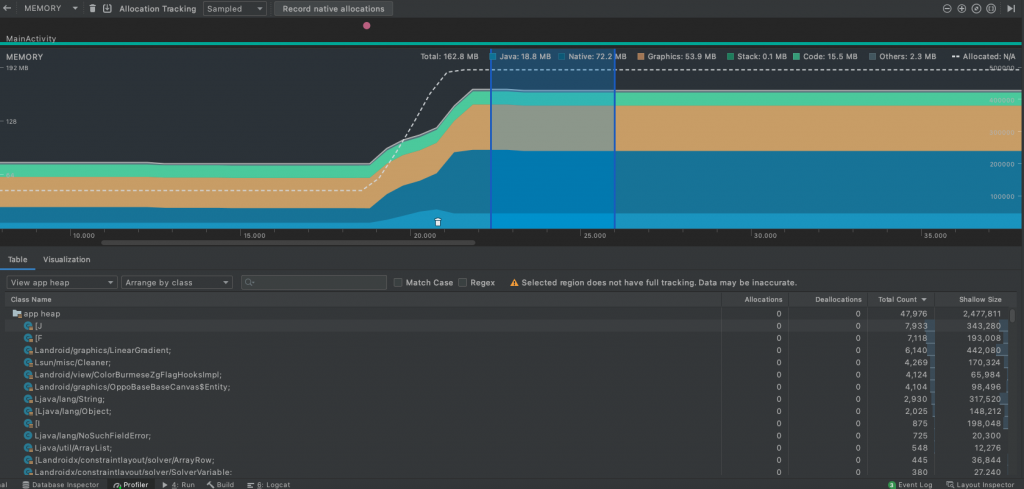#0

## 效率比較## 實際優化方案

### 實作

``````int* color0 = new int;
color0 = *tmpColor >> 16 & 0xff;
color0 = *tmpColor >> 8 & 0xff;
color0 = *tmpColor & 0xff;
``````
``````//整張圖的尺寸
int* tmpArray = new int[width * height];
for(int j = 0; j < width; j++) {
//漸變點的座標
int centerPosition = ((float) height)  * fullPositions[j];
for (int i = 0; i < height; i++) {
int red, green, blue;
//計算每個Pixel顏色
if(i < centerPosition) {
float ratio = (float)i / (float)centerPosition;
red = color0 + ((float)(color1 - color0) * ratio);
green = color0 + ((float)(color1 - color0) * ratio);
blue = color0 + ((float)(color1 - color0) * ratio);
}
else{
int secondHalf = height - centerPosition;
float ratio = (float)(i - centerPosition) / (float)secondHalf;
red = color1 + ((float)(color2 - color1) * ratio);
green = color1 + ((float)(color2 - color1) * ratio);
blue = color1 + ((float)(color2 - color1) * ratio);
}
tmpArray[i * width + j] = 255 << 24 | (red << 16) | (green << 8) | blue;
}
}
``````

``````Bitmap bmp = Bitmap.createBitmap(tmpArray, width, height, Bitmap.Config.ARGB_8888);
canvas.drawBitmap(bmp, 0, 0, paint);
``````